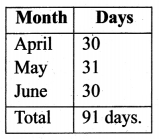## Tamilnadu Samacheer Kalvi 6th Maths Solutions Term 2 Chapter 2 Measurements Intext Questions

Try These (Textbook Page No. 26)

Question 1.
Complete the following table: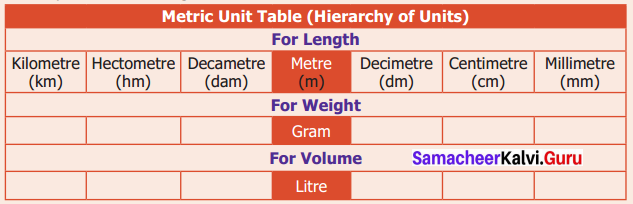Solution: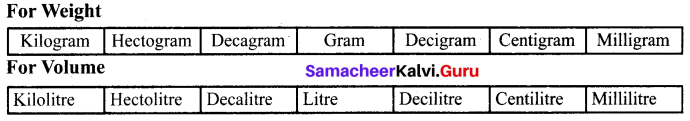Question 2.
Determine which metric unit you would use to express the following:

1. The length of your middle finger.
2. The weight of an elephant.
3. The weight of the ring.
4. The weight of the tablet.
5. The length of the safety pin.
6. The height of the building.
7. The length of the seashore in Tamilnadu.
8. The volume of a cup of coffee.
9. The capacity of water in the tank.

Solution:

1. Cm
2. Kilogram
3. gram
4. milligram
5. millimetre
6. metre
7. Kilometre
8. Millilitre
9. LitreTry These (Textbook Page No. 28)

Question 1.
Convert the following.
(i) 23 km into m
(ii) 1.78 m into cm
(iii) 7814 m into km
(iv) 8.67 mm into cm
(v) 16 l into ml
(vi) 1500 ml into l
(vii) 2360 l into kl
(viii) 873 l into ml
(ix) 40 mg into g
(x) 1550 g into kg
(xi) 6.5 kg into g
(xii) 723 g into mg
Solution: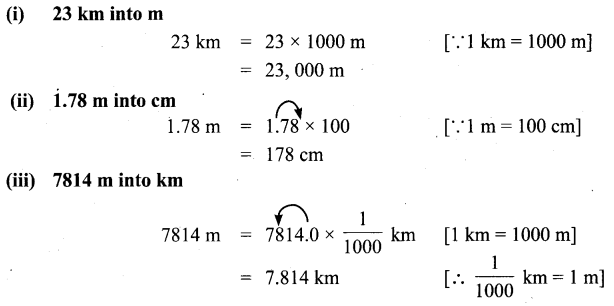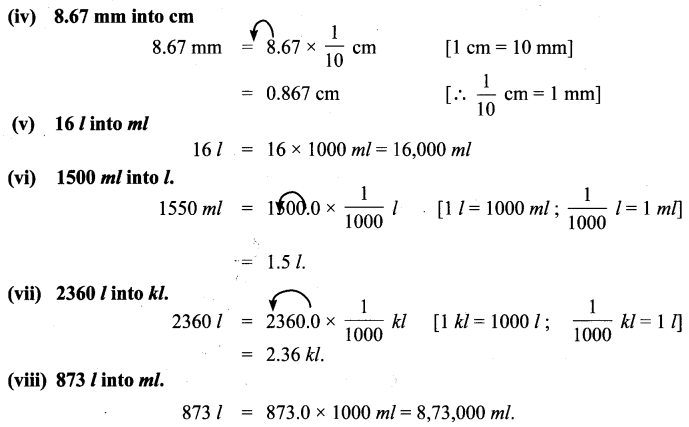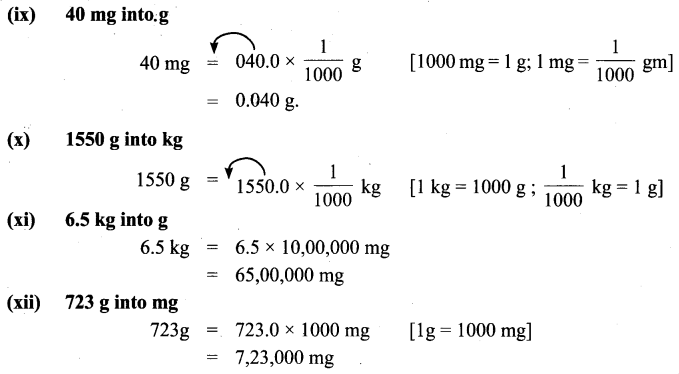Try These (Textbook Page No. 30)

Question 1.
Five kilograms of compost is needed for a coconut tree for every six months. How many kilograms of compost is needed for 50 such coconut trees for one and a half years?
Solution:
Compost needed for a tree for 6 months = 5 kg
Compost needed for 50 trees for half a year = 5 × 50 = 250 kg.
Compost needed for 1$$\frac { 1 }{ 2 }$$ years = 250 × 3 = 750 kg. [∴ 1$$\frac { 1 }{ 2 }$$ years = 3 half years]

Question 2.
Say True or False and Justify.
(a) Is it correct: 4 m + 3 cm = 7 m
(b) Can we add the following?
(i) 6 litre + 7 kg
(ii) 3 m + 5 l
(iii) 400 ml + 300 g
Solution:
(a) False.
4 m + 3 cm = 400 cm + 3 cm = 403 cm.
(b) (i) No, we can’t add.Recap (Textbook Page No. 33)

Question 1.
Read and write the time in the appropriate place.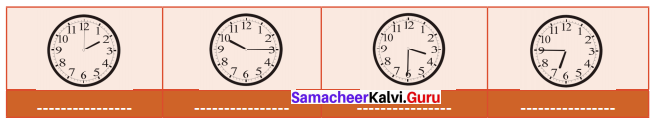Solution: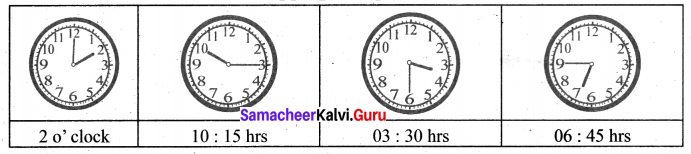Try These (Textbook Page No. 33)

Question 1.
Say the following time in two ways.
(a) 9.20
(b) 4.50
(c) 5.15
(d) 6.45
(e) 11.30
Solution:
(a) 9.20
20 minutes past 9
40 minutes to 10
(b) 4.50
50 minutes past 4
10 minutes to 5
(c) 5.15
15 minutes past 5
45 minutes to 6
(d) 6.45
45 minutes past 6
15 minutes to 7
(e) 11.30
30 minutes past 11
30 minutes to 12

Try These (Textbook Page No. 34)

Question 1.
Convert the following:
(i) 4 hours = _____ minutes
(ii) 240 minutes = ____ hours
(iii) 30 minutes = _____ seconds
(iv) 3600 seconds = _____ hours
(v) 2 hours = _____ seconds
Solution:
(i) 4 hours = 240 minutes
4 hours = 4 × 60 minutes = 240 minutes
(ii) 240 minutes = 4 hrs
240 minutes = $$\frac{240}{60}$$ = 4 hrs
(iii) 30 minutes = 1800 seconds
30 minutes = 30 × 60 seconds = 1800 seconds
(iv) 3600 seconds = 1 hrs
3600 seconds = $$\frac{3600}{60}$$ minutes = 60 minutes = $$\frac{60}{60}$$ hours = 1 hour
(v) 2 hrs = 7200 seconds
2 hrs = 2 × 60 minutes = 120 minutes = 120 × 60 seconds = 7200 secondsTry These (Textbook Page No. 36)

Question 1.
Convert the 12-hour format into the 24-hour format and vice versa.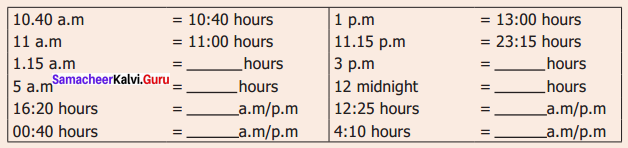Solution:
10 : 40 a.m. = 10 : 40 hours
11 a.m. = 11 : 00 hours
1 : 15 a.m. = 01 : 15 hours
5 a.m. = 05 : 00 hours
16 : 20 hours = 4 : 20 p.m.
00 : 40 hours = 12 :40 a.m.
1 p.m. = 13 : 00 hours
11 : 15 p.m. = 23 : 15 hours
3 p.m. = 15 :00 hours
12 midnight = 00 : 00 hours
12 : 25 hrs = 00 : 25 p.m.
4 : 10hrs = 4 : 10 a.m.

Try These (Textbook Page No. 39)

Question 1.
Check whether the following years as Ordinary or Leap Year?
1994; 1985; 2000; 2007; 2010; 2100
Solution:
We know that a leap year is divisible by 4.
In the case of a century which is divisible by 400 is a leap year.
1994 – Not a leap year (Not divisible by 4)
1985 – Not a leap year (Not divisible by 4)
2000 – It is a leap year (divisible by 400)
2007 – Not a leap year (Not divisible by 4)
2010 – Not a leap year (Not divisible by 4)
2100 – Not a leap year (Not divisible by 400)

Question 2.
How many days are there from 1st April to 30th June?
Solution:
Months from April to June are: